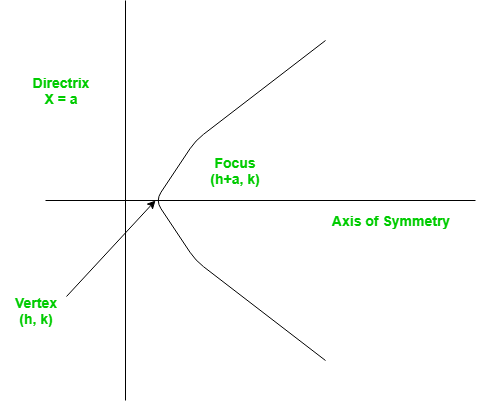# Python Program for Finding the vertex, focus and directrix of a parabola

python tutorials and learn python

# Python Program for Finding the vertex, focus and directrix of a parabola

A set of points on a plain surface that forms a curve such that any point on that curve is equidistant from the focus is a parabola.
Vertex of a parabola is the coordinate from which it takes the sharpest turn whereas a is the straight line used to generate the curve.The standard form of a parabola equation is. Given the values of a, b and c; our task is to find the coordinates of vertex, focus and the equation of the directrix.

Example –

```Input : 5 3 2
Output : Vertex:(-0.3, 1.55)
Focus: (-0.3, 1.6)
Directrix: y=-198
Consult the formula below for explanation.```
 `# Python program to calculate Vertex, Focus and Directrix `` ``def` `parabola(a, b, c): ``    ``print` `(``"Vertex: ("` `, (``-``b ``/` `(``2` `*` `a)) , ``", "``        ``,(((``4` `*` `a ``*` `c) ``-` `(b ``*` `b)) ``/` `(``4` `*` `a)) , ``")"` `) ``         ``    ``print` `(``"Focus: ("` `, (``-``b ``/` `(``2` `*` `a)) , ``", "``        ``, (((``4` `*` `a ``*` `c) ``-` `(b ``*` `b) ``+` `1``) ``/` `(``4` `*` `a)) , ``")"` `) ``     ``    ``print` `(``"Directrix: y="``            ``, (``int``)(c ``-` `((b ``*` `b) ``+` `1``) ``*` `4` `*` `a ))     ``     ``     ``# main() ``a ``=` `5``b ``=` `3``c ``=` `2`` ``parabola(a, b, c) `` ``# `

Output :

```Vertex:(-0.3, 1.55)
Focus: (-0.3, 1.6)
Directrix: y=-198```